## Tubing Length Change due to Piston Effect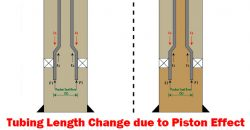This article demonstrates a piston effect and how it will change the length of tubing with full detailed calculations.

For the analysis, Lubinski’s sign conventions are used.

Compression force = ⇑(+)

Tensile force = ⇓ (-)

Shorten in Length = (-)

Elongate in length = (+)

Note: The tubing is free to move.

Please see this in the reference section to get a full paper.

Total force change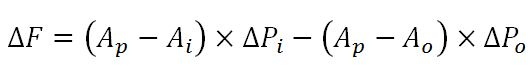Total length change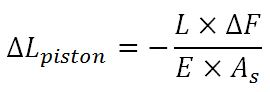Continue reading

## Piston Effect on Tapered String and Expansion Device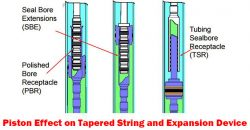In many situations, a completion string may consist of several size of strings and components so total axial force must be carefully considered by applying a free body diagram concept. When the tubing string is free to move, forces will affect the tubing above where the force is applied.

Tapered String

Tapered string is a string which consists of more than one size of tubing. Hence, forces acting at tapers must be accounted for. Force depends on configurations of pipe and pressure and it will either decrease or increase surface load.

Figure 1 illustrates diagram of a tapered completion string. Wherever in the string there is a difference in outside diameter or inside diameter, there will be downwards or upwards force acting against the tapered edge. It is imperative to analyze each ledge and combine all the results in order to see the total effect. From Figure 1, F1 and F2 cause compression, but F3 creates tension force.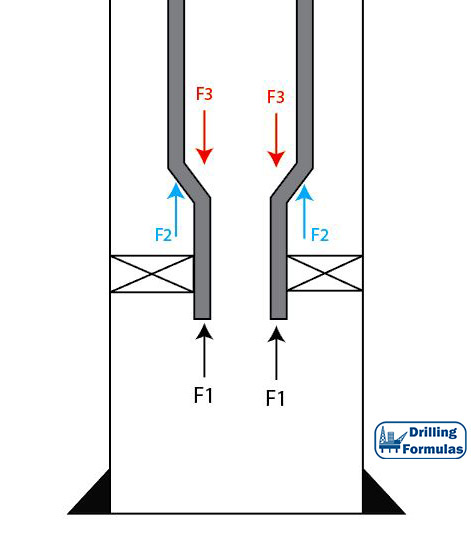Figure 1 – Tapered String Configuration

## Piston Force on Closed-Ended Tubular (Plugged Tubular)According to the previous post (piston force on open-ended tubular), applied surface pressure will reduce tensile force on surface. In this article, this is an analysis on the piston force on a plugged tubular string and the details are shown below;

Tubing Detail

• 5” Tubing
• ID of tubing = 3.696”
• Packer seal bore OD = 5.25”
• Weight per length = 17.7 lb/ft
• Total Length = 10,000 ft
• Plugged tubing depth = 10,000 ft
• Fluid density = 10 ppg
• Tubing is free to move in the packer
• Applied surface pressure = 5,000 psi

Figure 1 shows the wellbore schematic. Applied pressure (5,000 psi) will cause a piston effect to push the tubing. Therefore, at the bottom of tubing buoyancy and piston force will act in an upwards direction (compression).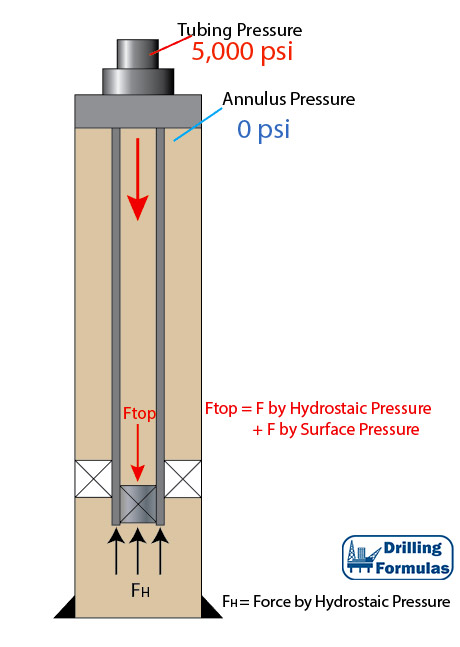Figure 1 – Wellbore Schematic

## Piston Force on Open-Ended TubularPiston force is a load caused directly by changes in pressure acting on the exposed cross sectional area of pipe. This results in changing in length of tubular and force acting against tubular. In this article, it generally demonstrates force distribution based on a simple tubular diagram.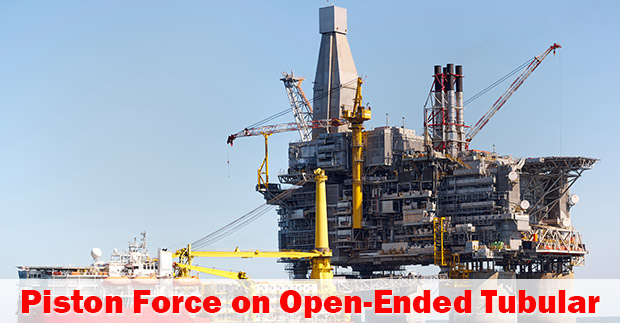Tubing Detail

• 5” Tubing
• ID of tubing = 3.696”
• Weight per length = 17.7 lb/ft
• Total Length = 10,000 ft
• Fluid density = 10 ppg
• Tubing is free to move in the packer
• Applied surface pressure = 5,000 psi